Courses

# Test: NEET Previous Year Questions: Work, Energy & Power- 2

## 9 Questions MCQ Test Physics Class 11 | Test: NEET Previous Year Questions: Work, Energy & Power- 2

Description
This mock test of Test: NEET Previous Year Questions: Work, Energy & Power- 2 for JEE helps you for every JEE entrance exam. This contains 9 Multiple Choice Questions for JEE Test: NEET Previous Year Questions: Work, Energy & Power- 2 (mcq) to study with solutions a complete question bank. The solved questions answers in this Test: NEET Previous Year Questions: Work, Energy & Power- 2 quiz give you a good mix of easy questions and tough questions. JEE students definitely take this Test: NEET Previous Year Questions: Work, Energy & Power- 2 exercise for a better result in the exam. You can find other Test: NEET Previous Year Questions: Work, Energy & Power- 2 extra questions, long questions & short questions for JEE on EduRev as well by searching above.
QUESTION: 1

### An ideal spring with spring-constant k is hung from the ceiling and a block of mass M is attached to its lower end. The mass is released with the spring initially unstretched. Then the maximum extension in the spring is

Solution:

Once the mass is released, the spring would extend Mg/k length where it comes to an equilibrium, but by then the mass would have gained some speed due to which the spring will be stretched Mg/k length more and then start a damped oscillation. Thus maximum extension by spring is 2Mg/k length.

QUESTION: 2

Solution:
QUESTION: 3

### In a region of only gravitational field of mass `M' a particle is shifted from A to B via three different paths in the figure. The work done in different paths are W1, W2, W3 respectively then [JEE(Scr.)-2003]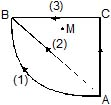Solution:

Since gravitational force is conservative in nature, so, only initial and final position matter.

QUESTION: 4

A particle is placed at the origin and a force F = kx is acting on it (where k is a positive constant). If U(0) = 0, the graph of U(x) versus x will be (where U is the potential energy function)

[JEE(Scr.)-2004]

Solution: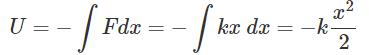This is the equation of parabola symmetric to U axis in negative direction.

QUESTION: 5

A bob of mass M is suspended by a massless string of length L. The horizontal velocity V at position A is just sufficient to make it reach the point B. The angle q at which the speed of the bob is half of that at A, satisfies

Solution: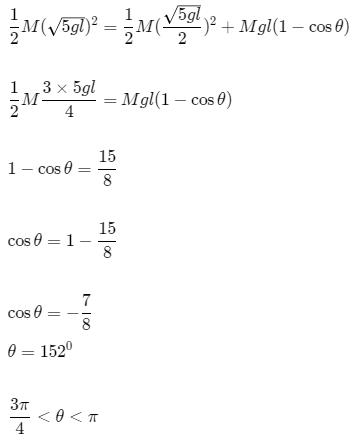QUESTION: 6

A light inextensible string that goes over a smooth fixed pulley as shown in the figure connects two blocks of masses 0.36 kg and 0.72 kg. Taking g = 10 m/s2, find the work done (in joules) by the string on the block of mass 0.36 kg during the first second after the system is released from rest.Solution:

2mg − T = (2m)a
T − mg = ma
⇒ a = g/3
T = 4mg/3
w = T × s
= T × 1/2at2
= 4/3mg * 1/2 * g/3 * 12
= 200/9 * 0.36
= 8 Joules
​

QUESTION: 7

A ball of mass (m) 0.5 kg is attached to the end of a string having length (L) 0.5 m. The ball is rotated on a horizontal circular path about vertical axis. The maximum tension that the string can bear is 324 N. the maximum possible value of angular velocity of ball (in radian/s) is

[jee-2011]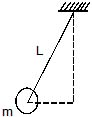Solution:

Forum id- 1557839
We have,
Tsinθ=mrω2
But,
sinθ=Lr​
∴ T=mLω2
∴ 324=0.5×0.5×ω2

*Answer can only contain numeric values
QUESTION: 8

A block of mass 0.18 kg is attached to a spring of force-constant 2 N/m. The coefficient of friction between the block and the floor is 0.1. Initially the block is at rest and the spring is un-stretched. An impulse is given to the block as shown in the figure. The block slides a distance of 0.06 m and comes to rest for the first time. The initial velocity of the block in m/s is V = N/10. Then N is

[JEE-2011]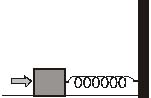Solution:
QUESTION: 9

Two identical discs of same radius R are rotating about their axes in opposite directions with the same constant angular speed w. The discs are in the same horizontal plane At time t = 0, the points P and Q are facing each other as shown in the figure. The relative speed between the two points P and Q is nr.In one time period (T) of rotation of the discs, nr as a function of time is best represented by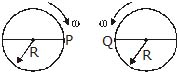[JEE-2012]

Solution:

Draw tangents to the circles at P and Q and at the top and bottom of the circles and mark the direction of motion on each.
As, both speeds are equal and at P and Q, the direction of motion is the same, relative speed is 0.

At the top and bottom of the path, the direction of motion of each of them is opposite to that of the other. Thus, relative speed increases.
At the diametrically opposite positions to P and Q, again the motion is in the same direction and the speeds are equal, so, relative speed is 0.
Thus, the relative speed increases from 0 to a maximum value as the discs rotate from P and Q to the bottom of the path , then it decreases to 0 as they rotate from the bottom to the diametrically opposite positions to P and Q , and again increases as they rotate to the top and finally becomes 0 as they come back again to their original position.